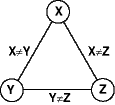Constraint Guide - Binarization

 Guide to Constraint Programming © Roman Barták, 1998 Contents Next

Binarization of Constraints

Most algorithms of constraint satisfaction restrict to the CSPs in which each constraint is either unary or binary. Such CSP is usually referred as a binary CSP.

Consequently, a binary CSP can be depicted by a constraint graph (sometimes referred as a constraint network), in which each node represents a variable, and each arc represents a constraint between variables represented by the end points of the arc. A unary constraint is formally represented by an arc originating and terminating at the same node. Clearly, the unary constraint can be immediately satisfied by reducing the domain of the constrained variable (node consistency), and thus, arcs representing unary constraints can be removed from the constraint network to simplify the constraint satisfaction algorithms.

 Example: constraint system: X#Y Y#Z X#Z constraint network:Because it is possible to convert any CSP with n-ary constraints to another equivalent binary CSP, the restriction to binary CSPs is not limitative at all.

The conversion of arbitrary CSP to an equivalent binary CSP is based on the idea of introducing a new variable that encapsulates the set of constrained variables. This newly introduced variable, we call it an encapsulated variable, has assigned a domain that is a Cartesian product of the domains of individual variables. Note, that if the domains of individual variables are finite than the Cartesian product of the domains, and thus the resulting domain, is still finite.

Example:

original (individual) variables and their domains:

X::[1,2]
Y::[3,4]
Z::[5,6]

encapsulated variable and its domain:

 U:: [(1,3,5),(1,3,6),  (1,4,5),(1,4,6),  (2,3,5),(2,3,6)  (2,4,5),(2,4,6)]

Now, arbitrary n-ary constraint can be converted to equivalent unary constraint that constrains the variable which appears as an encapsulation of the original individual variables. As we mentioned above, this unary constraint can be immediately satisfied by reducing the domain of encapsulated variable. Briefly speaking, n-ary constraint can be substituted by an encapsulated variable with the domain corresponding to the constraint.

 Example: original constraint and variables: X+Y=Z X::[1,2]; Y::[3,4]; Z::[5,6] encapsulated variable and reduced domain: U::[(1,4,5),(2,3,5),(2,4,6)]

In fact, this transformation solves individual constraints. Now, it remains to combine these individual solutions to the solution of the constraint system. There are two ways how to bind the encapsulated variables:With original variables (hidden variable encoding)

Combination of the original and encapsulated variables naturally expresses the original non-binary CSP as the encapsulated variables directly correspond to the original constraints. Newly introduced constraints just bind the original and encapsulated variables in a following way:

X=i_th_argument_of(U),

where X is the original variable, U is the encapsulated variable and i is the "position of X within U".

 Example: original (non-binary) CSP: X+Y=Z, X

The advantage of this approach is the preservation of the original variables, so the solution of the original CSP can be directly obtained from the solution of the binary CSP (the evaluation of the encapsulated variables is omitted). Another advantage is the preservation of the binary constraints from the original CSP.Without original variables (dual encoding)

The other approach to represent converted binary CSP is based on using encapsulated variables only. Then, the newly introduced constraints just binds these variables via common components in a following way"

i_th_argument_of(U)=j_th_argument_of(V),

where U and V are encapsulated variables and i and j respectively are the "positions of common component".

 Example: original (non-binary) CSP: X+Y=Z, X

In this approach, each constraint from the original CSP is represented by an encapsulated variable. The resulting constraint network is smaller than the network constructed by the previous method, however, the solution, i.e., the valuation of original variables, has to be extracted from the valuation of encapsulated variables.

The possibility to express constraints of higher arity in terms of binary constraints is important from the theoretical point of view as it enables us to restrict to binary CSPs. Hence, in some sence, binary CSPs are representative of all CSPs. Nevertheless, in practice the binarization is not likely to be worth doing.

On the equivalence of constraint satisfaction problems,
F. Rossi, V. Dahr and C. Petrie, in Proc. European Conference on Artificial Intelligence (ECAI-90), Stockholm, 1990. Also MCC Technical Report ACT-AI-222-89.

On the conversion between Non-Binary and Binary Constraint Satisfaction Problems,
F. Bacchus, P. van Beek, in Proc. National Conference on Artifical Intelligence (AAAI-98), Madison, Wisconsin, 1998.

Encodings of Non-Binary Constraint Satisfaction Problems,
K. Stergiou, T. Walsh, in Proc. National Conference on Artifical Intelligence (AAAI-99), Orlando, Florida, 1999.

Using auxiliary variables and implied constraints to model non-binary problems,
B. Smith, K. Stergiou, T. Walsh, in Proc. National Conference on Artifical Intelligence (AAAI-00), Austin, Texas, 2000.

Non-Binary Constraints,
C. Bessiere, in Proc. Principles and Practice of Constraint Programming (CP-99), Alexandria, Virginia, USA, 1999.

 Contents Next Designed and maintained by Roman Barták